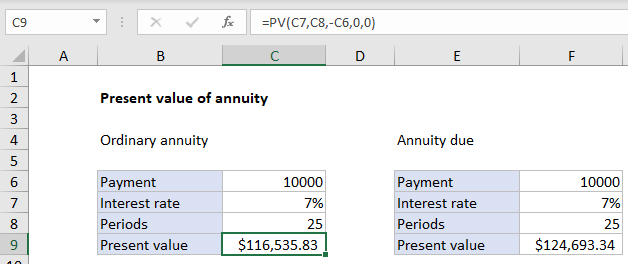# How to calculate present value of annuity in excel

To get the present value of an annuity, you can use the PV function.

## Formula

`=PV(rate,periods,payment,0,0)`

In the example shown, the formula in C9 is:

`=PV(C5,C6,C4,0,0)`### Explanation

An annuity is a series of equal cash flows, spaced equally in time. In this example, an annuity pays 10,000 per year for the next 25 years, with an interest rate (discount rate) of 7%. To calculate present value, the PV function is configured as follows:

• nper – the value from cell C8, 25.
• pmt – the value from cell C6, 100000.
• rate – the value from cell C7, 7%.
• fv – 0.
• type – 0, payment at end of period (regular annuity).
Worked Example:   Calculate original loan amount in Excel

With this information, the present value of the annuity is \$116,535.83. Note payment is entered as a negative number, so the result is positive.

Worked Example:   PMT, RATE, NPER, PV and FV Financial Functions in Excel

### Annuity due

With an annuity due, payments are made at the beginning of the period, instead of the end. To calculate present value for an annuity due, use 1 for the type argument. In the example shown, the formula in F9 is:

`=PV(F7,F8,-F6,0,1)`

Note the inputs (which come from column F) are the same as the original formula. The only difference is type = 1.